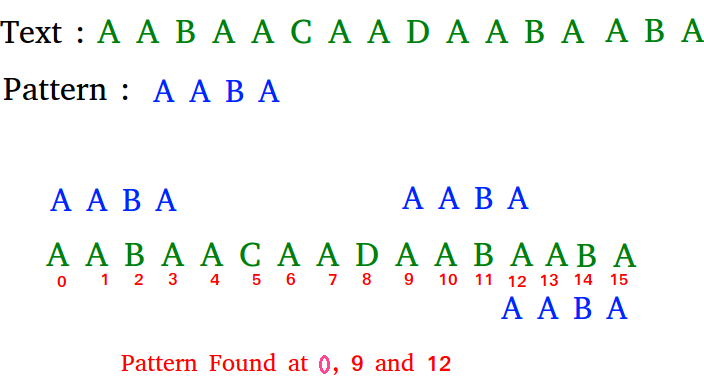# C Program for Naive algorithm for Pattern Searching

Given a text txt[0..n-1] and a pattern pat[0..m-1], write a function search(char pat[], char txt[]) that prints all occurrences of pat[] in txt[]. You may assume that n > m.

Examples:

```Input:  txt[] = "THIS IS A TEST TEXT"
pat[] = "TEST"
Output: Pattern found at index 10

pat[] =  "AABA"
Output: Pattern found at index 0
Pattern found at index 9
Pattern found at index 12```

Pattern searching is an important problem in computer science. When we do search for a string in notepad/word file or browser or database, pattern searching algorithms are used to show the search results.

## C

 `// C program for Naive Pattern Searching algorithm ` `#include ` `#include ` ` `  `void` `search(``char``* pat, ``char``* txt) ` `{ ` `    ``int` `M = ``strlen``(pat); ` `    ``int` `N = ``strlen``(txt); ` ` `  `    ``/* A loop to slide pat[] one by one */` `    ``for` `(``int` `i = 0; i <= N - M; i++) { ` `        ``int` `j; ` ` `  `        ``/* For current index i, check for pattern match */` `        ``for` `(j = 0; j < M; j++) ` `            ``if` `(txt[i + j] != pat[j]) ` `                ``break``; ` ` `  `        ``if` `(j == M) ``// if pat[0...M-1] = txt[i, i+1, ...i+M-1] ` `            ``printf``(``"Pattern found at index %d \n"``, i); ` `    ``} ` `} ` ` `  `/* Driver program to test above function */` `int` `main() ` `{ ` `    ``char` `txt[] = ``"AABAACAADAABAAABAA"``; ` `    ``char` `pat[] = ``"AABA"``; ` `    ``search(pat, txt); ` `    ``return` `0; ` `} `

Output:

```Pattern found at index 0
Pattern found at index 9
Pattern found at index 13
```

Please refer complete article on Naive algorithm for Pattern Searching for more details!

My Personal Notes arrow_drop_up
Article Tags :

Be the First to upvote.

Please write to us at contribute@geeksforgeeks.org to report any issue with the above content.